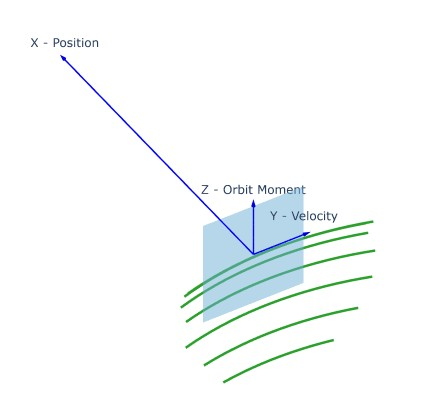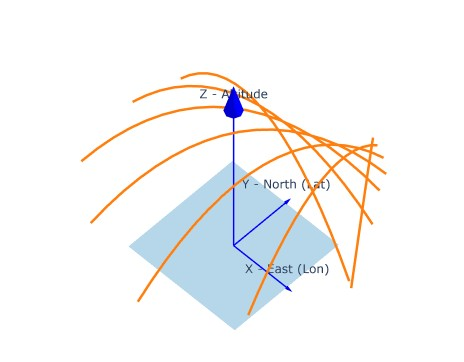# Topocentric Frame Conversion?

Hi Everyone,

I’ve run into a problem with frame transformation which makes me wonder if I conceptually misunderstood something. Maybe you can help? I’d like the AzEl of a satellite vector in the Topocentric Frame of a ground station. I tried two different methods, but they don’t agree and I don’t understand why.

Scenario setup:

``````# Define Earth & Time
ECI = FramesFactory.getEME2000()
ECF = FramesFactory.getITRF(IERSConventions.IERS_2010, True)
Earth = OneAxisEllipsoid( ..., ECF)
time = AbsoluteDate(...)

# Define Satellite
sat_orbit = KeplerianOrbit(a,e,i,w,r,n,PositionAngle.TRUE,ECI,epoch,mu)
sat_propg = KeplerianPropagator(sat_orbit)

sat_state = sat_propg.propagate(time)
sat_pvc_eci = sat_state.getPVCoordinates()
sat_vec_eci = sat_pvc_eci.position

# Define Location
loc_point = GeodeticPoint(lat,lon,alt)
loc_vec_ecf = Earth.transform(loc_point)
loc_vel_ecf = Vector3D(0.0, 0.0, 0.0)

loc_pvc_ecf = AbsolutePVCoordinates(ECF, time, pos, vel)  # 'state'
loc_pvc_eci = loc_pvc_ecf.getPVCoordinates(time, ECI)
loc_vec_eci = lc_pvc_eci.position
``````

The two AzEl Methods:

``````# AzEl (Method 1)
loc_frame = TopocentricFrame(Earth, point, "local frame")
elv = loc_frame.getElevation(sat_vec_eci, ECI, time)
azm = loc_frame.getAzimuth(sat_vec_eci, ECI, time)

# AzEl (Method 2)
eci_to_topo = ECI.getTransformTo(loc_frame, time)
sat_pvc_loc = eci_to_topo.transformPVCoordinates(sat_pvc_eci)
sat_vec_loc = sat_pvc_loc.position

elv = sat_vec_loc.delta # <<-- same as above, ok
azm = sat_vec_loc.alpha # <<-- 90 deg offset, why??

``````

Hi there,

There are several conventions to define azimuth. From the top of my mind, Orekit’s one is to measure it from the north pointing vector. So it’s not equal to the so called alpha angle of Hipparchus’ `Vector3D` in the topocentric frame.

Best,
Romain.

Hi Romain, thanks for the comment. I looked at the source of the TopocentricFrame.getElevation() / getAzimuth() methods, and you’re right they use different definitions than the Vector3D.delta / .alpha attributes. In the end, I used my own calculation instead - below in case this is helpful to anybody:

``````elv = asin(vec.x/vec.norm)
azm = atan2(vec.y, vec.z)
``````

This yields elevation above/below the (y,z) plane, and azimuth around the x-axis, from the position vector of the object being viewed in the same (local) frame.

Hum the thing is that in Orekit’s topocentric frame, Z is the zenith direction, so your code doesn’t yield an elevation (a.k.a. altitude) as understood for angular measurements. As hinted in your first post, elevation actually is `Vector3D`’s delta.

Romain.

Agree, as you said it depends on how (relative to what plane) you define it. The equations above are for az/el relative to the y/z plane, with the y-axis marking zero azimuth. In the Topocentric frame as Orekit uses it, it’s the x/y plane, with x pointing north defining the zero azm angle.

I’ve used this for both a ground station (Topocentric, Az/El relative to x/y plane) and a satellite (Local Vertical Local Horizontal frame, AzEl in y/z plane) and the results look consistent.